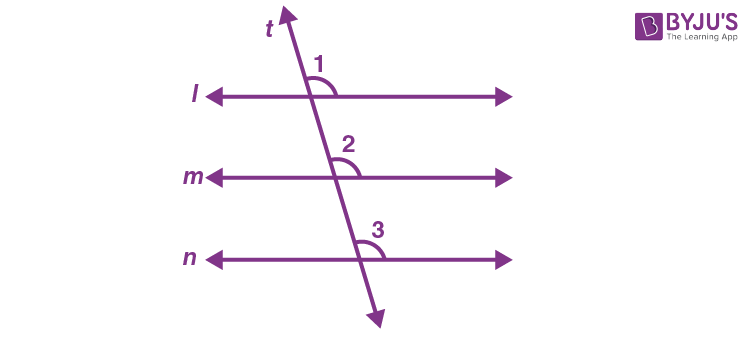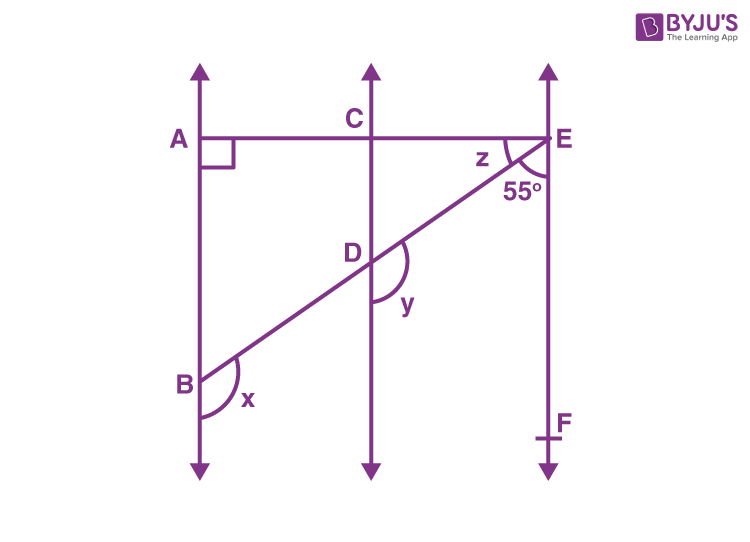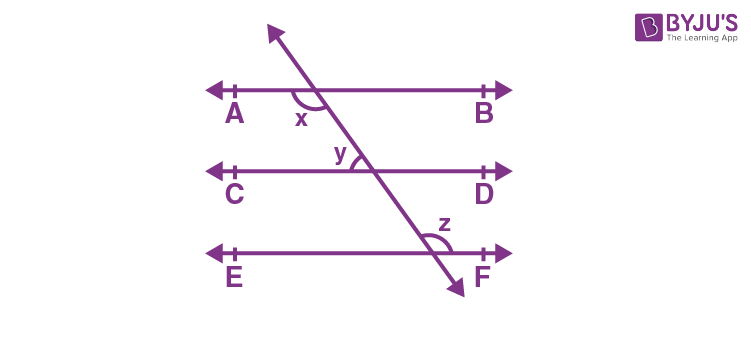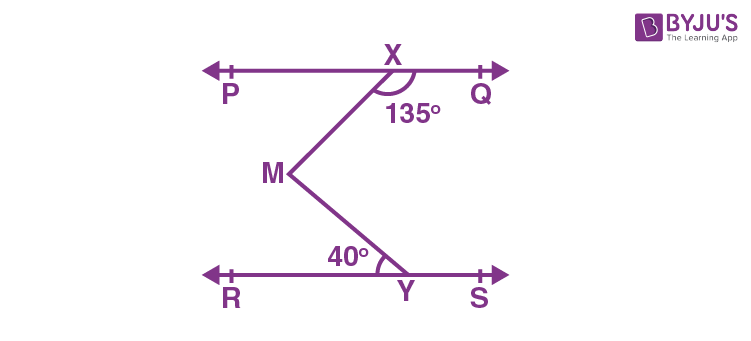# Lines Parallel to the Same Line

In Geometry, a line is defined as a one-dimensional geometric figure that extends infinitely in both directions. A line has no thickness. A line is generally made up of an infinite number of points. In this article, we will discuss the lines parallel to the same line and the theorem related to it with many solved examples.

## What is Meant by Lines Parallel to the Same Line?

Theorem: Lines that are parallel to the same line are parallel to each other.

It means that if two lines are parallel to the same line, then they will be parallel to each other. Now, let us check this theorem with the help of the below figure.From the given figure, we can say that line m is parallel to line l and line n is parallel to line l. (i.e) line m || line l and line n || line l. Also, “t” is the transversal for the lines l, m and n.

Therefore, we can say that ∠1 = ∠2 and ∠1 = ∠3. (By corresponding angles axioms).

So, we can also say that ∠2 and ∠3 are corresponding and they are equal to each other.

Thus, ∠2 = ∠3

By using the converse of corresponding angle axioms, we conclude that line m is parallel to line n.

(i.e) Line m || Line n.

Note: This property can be extended to more than two lines also.

### Solved Example

Example:

From the given figure, AB is parallel to CD and CD is parallel to EF.Also, given that EA is perpendicular to AB. Find the values of x, y and z, if ∠BEF = 55°.

Solution:

Given that AB|| CD, CD || EF, EA ⊥ AB, and ∠BEF = 55°.

Therefore, y+ 55° = 180° (Interior angles on the same side of transversal ED)

Hence, y = 180°-55°= 125°

By using the corresponding angles axiom, AB || CD, we can say that x = y.

Therefore, the value of x= 125°.

Since, AB || CD and CD || EF, therefore AB || EF.

So, we can write: ∠ FEA + ∠ EAB = 180° (Interior angles on the same side of transversal EA)

55° + z + 90° = 180°

z = 180° – 90° – 55°

z = 180° – 145°

z = 35°

Therefore, the values of x, y and z are 125°, 125° and 35°, respectively.

### Practice Problems

Solve the following problems:

1. From the given figure, AB ||CD and CD || EF and y:z = 3:7. Find the value of x.2. From the given figure, if PQ || RS and ∠MXQ = 135° and ∠MYR = 40°, then find the value of ∠XMY.Stay tuned with BYJU’S – The Learning App and learn more class-wise concepts easily by exploring more videos.

## Frequently Asked Questions on Line Parallel to the Same Line

### What is meant by a line parallel to the same line?

The lines which are parallel to the same line are parallel to each other.

### Which symbol is used to represent the parallel line?

The symbol used to represent the parallel line is “||”.

### What is meant by parallel lines?

The parallel lines are the lines that are equidistant from each other and they never intersect each other.

### Mention three properties of angles associated with parallel lines.

The corresponding angles are equal
Alternate interior angles are equal
Alternate exterior angles are equal.

### How do you prove that the given lines are parallel lines?

To prove that the given lines are parallel, then we have to prove either corresponding angles are equal or alternate angles are equal or co-interior angles are supplementary.